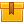# AC Characteristics (A8202)

## Prerequisites

This lesson is designed for participants familiar with Ohm's Law.

## Description

This lesson covers the basic characteristics of AC circuits including the relationship between voltage and current flow in an AC circuit, the way in which AC voltage is induced, and the cause and effect of inductance and capacitance in AC circuits. The lesson shows and explains how to use a sine wave to interpret changes in AC voltage over time, how to determine the frequency of AC voltage, and how to recognize the effects of inductance and capacitance in AC circuits.

## Objectives

State the basic operating characteristics of AC voltage. Use a sine wave to determine the frequency of AC voltage. Define the principles of magnetic attraction and repulsion. Define lines of flux and flux density. Describe how AC voltage is induced. Describe how a capacitor operates in an AC circuit. Describe how capacitance affects the relationship between voltage and current in an AC circuit. Interpret AC voltage over time.

## ContentThis lesson covers the basic characteristics of AC circuits including
the relationship between voltage and current flow in an AC circuit, the
way in which AC voltage is induced, and the cause and effect of
inductance and capacitance in AC circuits. The lesson shows and explains
how to use a sine wave to interpret changes in AC voltage over time,
how to determine the frequency of AC voltage, and how to recognize the
effects of inductance and capacitance in AC circuits.

•AC Characteristics

0
0 Reviews